# class TFoam: public TObject

```
FOAM  Version 1.02M

Authors:
Institute of Nuclear Physics, Cracow, Poland

What is FOAM for?

* Suppose you want to generate randomly points (vectors) according to
an arbitrary probability distribution  in n dimensions,
for which you supply your own subprogram. FOAM can do it for you!
Even if your distributions has quite strong peaks and is discontinuous!
* FOAM generates random points with weight one or with variable weight.
* FOAM is capable to integrate using efficient "adaptive" MC method.
(The distribution does not need to be normalized to one.)
How does it work?

FOAM is the simplified version of the multi-dimensional general purpose
Monte Carlo event generator (integrator) FOAM.
It creates hyper-rectangular "foam of cells", which is more dense around its peaks.
See the following 2-dim. example of the map of 1000 cells for doubly peaked distribution:

```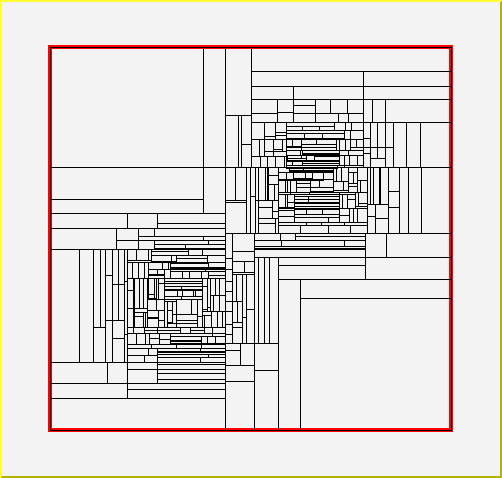``` FOAM is now fully integrated with the ROOT package.
The important bonus of the ROOT use is persistency of the FOAM objects!

For more sophisticated problems full version of FOAM may be more appropriate:

```
See full version of FOAM
``` Simple example of the use of FOAM:

Int_t kanwa(){
TH2D  *hst_xy = new TH2D("hst_xy" ,  "x-y plot", 50,0,1.0, 50,0,1.0);
Double_t *MCvect =new Double_t; // 2-dim vector generated in the MC run
TRandom3  *PseRan   = new TRandom3();  // Create random number generator
PseRan->SetSeed(4357);                // Set seed
TFoam   *FoamX    = new TFoam("FoamX");   // Create Simulator
FoamX->SetkDim(2);          // No. of dimensions, obligatory!
FoamX->SetnCells(500);      // No. of cells, can be omitted, default=2000
FoamX->SetRhoInt(Camel2);   // Set 2-dim distribution, included below
FoamX->SetPseRan(PseRan);   // Set random number generator
FoamX->Initialize();        // Initialize simulator, takes a few seconds...
// From now on FoamX is ready to generate events according to Camel2(x,y)
for(Long_t loop=0; loop<100000; loop++){
FoamX->MakeEvent();          // generate MC event
FoamX->GetMCvect( MCvect);   // get generated vector (x,y)
Double_t x=MCvect;
Double_t y=MCvect;
if(loop<10) cout<<"(x,y) =  ( "<< x <<", "<< y <<" )"<<endl;
hst_xy->Fill(x,y);           // fill scattergram
}// loop
Double_t mcResult, mcError;
FoamX->GetIntegMC( mcResult, mcError);  // get MC integral, should be one
cout << " mcResult= " << mcResult << " +- " << mcError <<endl;
// now hst_xy will be plotted visualizing generated distribution
TCanvas *cKanwa = new TCanvas("cKanwa","Canvas for plotting",600,600);
cKanwa->cd();
hst_xy->Draw("lego2");
}//kanwa
Double_t sqr(Double_t x){return x*x;};
Double_t Camel2(Int_t nDim, Double_t *Xarg){
// 2-dimensional distribution for FOAM, normalized to one (within 1e-5)
Double_t x=Xarg;
Double_t y=Xarg;
Double_t GamSq= sqr(0.100e0);
Double_t Dist=exp(-(sqr(x-1./3) +sqr(y-1./3))/GamSq)/GamSq/TMath::Pi();
Dist        +=exp(-(sqr(x-2./3) +sqr(y-2./3))/GamSq)/GamSq/TMath::Pi();
return 0.5*Dist;
}// Camel2
Two-dim. histogram of the MC points generated with the above program looks as follows:

```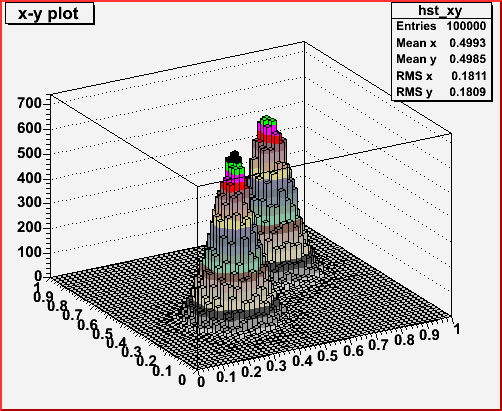``` Canonical nine steering parameters of FOAM

Name     | default  | Description

kDim     | 0        | Dimension of the integration space. Must be redefined!
nCells   | 1000     | No of allocated number of cells,
nSampl   | 200      | No. of MC events in the cell MC exploration
nBin     | 8        | No. of bins in edge-histogram in cell exploration
OptRej   | 1        | OptRej = 0, weighted; OptRej=1, wt=1 MC events
OptDrive | 2        | Maximum weight reduction, =1 for variance reduction
EvPerBin | 25       | Maximum number of the effective wt=1 events/bin,
|          | EvPerBin=0 deactivates this option
Chat     | 1        | =0,1,2 is the ``chat level'' in the standard output
MaxWtRej | 1.1      | Maximum weight used to get w=1 MC events

The above can be redefined before calling 'Initialize()' method,
for instance FoamObject->SetkDim(15) sets dimension of the distribution to 15.
Only kDim HAS TO BE redefined, the other parameters may be left at their defaults.
nCell may be increased up to about million cells for wildly peaked distributions.
Increasing nSampl sometimes helps, but it may cost CPU time.
MaxWtRej may need to be increased for wild a distribution, while using OptRej=0.

Past versions of FOAM: August 2003, v.1.00; September 2003 v.1.01
Adopted starting from FOAM-2.06 by P. Sawicki

Users of FOAM are kindly requested to cite the following work:
S. Jadach, Computer Physics Communications 152 (2003) 55.

```

## Function Members (Methods)

public:
 TFoam() TFoam(const Char_t*) TFoam(const TFoam&) virtual ~TFoam() void TObject::AbstractMethod(const char* method) const virtual void TObject::AppendPad(Option_t* option = "") virtual void TObject::Browse(TBrowser* b) void Carver(Int_t&, Double_t&, Double_t&) Int_t CellFill(Int_t, TFoamCell*) void CheckAll(Int_t) static TClass* Class() virtual const char* TObject::ClassName() const virtual void TObject::Clear(Option_t* = "") virtual TObject* TObject::Clone(const char* newname = "") const virtual Int_t TObject::Compare(const TObject* obj) const virtual void TObject::Copy(TObject& object) const virtual void TObject::Delete(Option_t* option = "") virtual Int_t TObject::DistancetoPrimitive(Int_t px, Int_t py) Int_t Divide(TFoamCell*) virtual void TObject::Draw(Option_t* option = "") virtual void TObject::DrawClass() const virtual TObject* TObject::DrawClone(Option_t* option = "") const virtual void TObject::Dump() const virtual void TObject::Error(const char* method, const char* msgfmt) const Double_t Eval(Double_t*) virtual void TObject::Execute(const char* method, const char* params, Int_t* error = 0) virtual void TObject::Execute(TMethod* method, TObjArray* params, Int_t* error = 0) virtual void TObject::ExecuteEvent(Int_t event, Int_t px, Int_t py) void Explore(TFoamCell* Cell) virtual void TObject::Fatal(const char* method, const char* msgfmt) const void Finalize(Double_t&, Double_t&) virtual TObject* TObject::FindObject(const char* name) const virtual TObject* TObject::FindObject(const TObject* obj) const void GenerCel2(TFoamCell*&) virtual Option_t* TObject::GetDrawOption() const static Long_t TObject::GetDtorOnly() virtual const char* TObject::GetIconName() const void GetIntegMC(Double_t&, Double_t&) void GetIntNorm(Double_t&, Double_t&) void GetMCvect(Double_t*) Double_t GetMCwt() void GetMCwt(Double_t&) virtual const char* TObject::GetName() const Long_t GetnCalls() const Long_t GetnEffev() const virtual char* TObject::GetObjectInfo(Int_t px, Int_t py) const static Bool_t TObject::GetObjectStat() virtual Option_t* TObject::GetOption() const Double_t GetPrimary() const void GetPrimary(Double_t& prime) TRandom* GetPseRan() const TFoamIntegrand* GetRho() virtual const char* TObject::GetTitle() const Int_t GetTotDim() const virtual UInt_t TObject::GetUniqueID() const const char* GetVersion() const void GetWtParams(Double_t, Double_t&, Double_t&, Double_t&) void Grow() virtual Bool_t TObject::HandleTimer(TTimer* timer) virtual ULong_t TObject::Hash() const virtual void TObject::Info(const char* method, const char* msgfmt) const virtual Bool_t TObject::InheritsFrom(const char* classname) const virtual Bool_t TObject::InheritsFrom(const TClass* cl) const void InitCells() void Initialize() void Initialize(TRandom*, TFoamIntegrand*) virtual void TObject::Inspect() const void TObject::InvertBit(UInt_t f) virtual TClass* IsA() const virtual Bool_t TObject::IsEqual(const TObject* obj) const virtual Bool_t TObject::IsFolder() const Bool_t TObject::IsOnHeap() const virtual Bool_t TObject::IsSortable() const Bool_t TObject::IsZombie() const void LinkCells() virtual void TObject::ls(Option_t* option = "") const void MakeActiveList() void MakeAlpha() void MakeEvent() void TObject::MayNotUse(const char* method) const Double_t MCgenerate(Double_t* MCvect) virtual Bool_t TObject::Notify() static void TObject::operator delete(void* ptr) static void TObject::operator delete(void* ptr, void* vp) static void TObject::operator delete[](void* ptr) static void TObject::operator delete[](void* ptr, void* vp) void* TObject::operator new(size_t sz) void* TObject::operator new(size_t sz, void* vp) void* TObject::operator new[](size_t sz) void* TObject::operator new[](size_t sz, void* vp) TFoam& operator=(const TFoam&) virtual void TObject::Paint(Option_t* option = "") Long_t PeekMax() virtual void TObject::Pop() virtual void TObject::Print(Option_t* option = "") const void PrintCells() virtual Int_t TObject::Read(const char* name) virtual void TObject::RecursiveRemove(TObject* obj) void TObject::ResetBit(UInt_t f) void ResetPseRan(TRandom* PseRan) void ResetRho(TFoamIntegrand* Rho) void RootPlot2dim(Char_t*) virtual void TObject::SaveAs(const char* filename = "", Option_t* option = "") const virtual void TObject::SavePrimitive(basic_ostream >& out, Option_t* option = "") void TObject::SetBit(UInt_t f) void TObject::SetBit(UInt_t f, Bool_t set) void SetChat(Int_t Chat) virtual void TObject::SetDrawOption(Option_t* option = "") static void TObject::SetDtorOnly(void* obj) void SetEvPerBin(Int_t EvPerBin) void SetInhiDiv(Int_t, Int_t) void SetkDim(Int_t kDim) void SetMaxWtRej(Double_t MaxWtRej) void SetnBin(Int_t nBin) void SetnCells(Long_t nCells) void SetnSampl(Long_t nSampl) static void TObject::SetObjectStat(Bool_t stat) void SetOptDrive(Int_t OptDrive) void SetOptRej(Int_t OptRej) void SetPseRan(TRandom* PseRan) void SetRho(TFoamIntegrand* Rho) void SetRhoInt(void* Rho) virtual void TObject::SetUniqueID(UInt_t uid) void SetXdivPRD(Int_t, Int_t, Double_t*) virtual void ShowMembers(TMemberInspector& insp, char* parent) virtual void Streamer(TBuffer& b) void StreamerNVirtual(TBuffer& b) virtual void TObject::SysError(const char* method, const char* msgfmt) const Bool_t TObject::TestBit(UInt_t f) const Int_t TObject::TestBits(UInt_t f) const virtual void TObject::UseCurrentStyle() void Varedu(Double_t*, Int_t&, Double_t&, Double_t&) virtual void TObject::Warning(const char* method, const char* msgfmt) const virtual Int_t TObject::Write(const char* name = 0, Int_t option = 0, Int_t bufsize = 0) virtual Int_t TObject::Write(const char* name = 0, Int_t option = 0, Int_t bufsize = 0) const
protected:
 virtual void TObject::DoError(int level, const char* location, const char* fmt, va_list va) const void TObject::MakeZombie()
private:
 Double_t Sqr(Double_t x) const

## Data Members

public:
 enum TObject::EStatusBits { kCanDelete kMustCleanup kObjInCanvas kIsReferenced kHasUUID kCannotPick kNoContextMenu kInvalidObject }; enum TObject::[unnamed] { kIsOnHeap kNotDeleted kZombie kBitMask kSingleKey kOverwrite kWriteDelete };
private:
 Double_t* fAlpha [fDim] Internal parameters of the hyperrectangle TFoamCell** fCells [fNCells] Array of ALL cells TRefArray* fCellsAct Array of pointers to active cells, constructed at the end of foam build-up Int_t fChat Chat=0,1,2 chat level in output, Chat=1 normal level TString fDate Release date of FOAM Int_t fDim Dimension of the integration/simulation space Int_t fEvPerBin Maximum number of effective (wt=1) events per bin TObjArray* fHistDbg Histograms of wt, for debug TObjArray* fHistEdg Histograms of wt, one for each cell edge TH1D* fHistWt Histogram of the MC wt Int_t* fInhiDiv ! [fDim] Flags for inhibiting cell division Int_t fLastCe Index of the last cell TFoamMaxwt* fMCMonit Monitor of the MC weight for measuring MC efficiency Double_t fMCerror and its error Double_t fMCresult True Integral R from MC series Double_t* fMCvect [fDim] Generated MC vector for the outside user Double_t fMCwt MC weight Int_t* fMaskDiv ! [fDim] Dynamic Mask for cell division Double_t fMaxWtRej Maximum weight in rejection for getting wt=1 events TMethodCall* fMethodCall ! ROOT's pointer to user-defined global distribution function Int_t fNBin No. of bins in the edge histogram for cell MC exploration Long_t fNCalls Total number of the function calls Int_t fNCells Maximum number of cells Long_t fNEffev Total number of effective events (wt=1) in the foam buildup Int_t fNSampl No. of MC events, when dividing (exploring) cell TString fName Name of a given instance of the FOAM class Double_t fNevGen Total number of the generated MC events Int_t fNoAct Number of active cells Int_t fOptDrive Optimization switch =1,2 for variance or maximum weight optimization Int_t fOptPRD Option switch for predefined division, for quick check Int_t fOptRej Switch =0 for weighted events; =1 for unweighted events in MC Double_t* fPrimAcu [fNoAct] Array of cumulative probability of all active cells Double_t fPrime Primary integral R' (R=R') TRandom* fPseRan Pointer to user-defined generator of pseudorandom numbers Int_t fRNmax Maximum No. of the rand. numb. requested at once TFoamIntegrand* fRho Pointer to the user-defined integrand function/distribution Double_t* fRvec [fRNmax] random number vector from r.n. generator fDim+1 maximum elements Double_t fSumOve Total Sum of overveighted events Double_t fSumWt Double_t fSumWt2 Total sum of wt and wt^2 TString fVersion Actual version of the FOAM like (1.01m) Double_t fWtMax Double_t fWtMin Maximum/Minimum MC weight TFoamVect** fXdivPRD ! Lists of division values encoded in one vector per direction

## Class Charts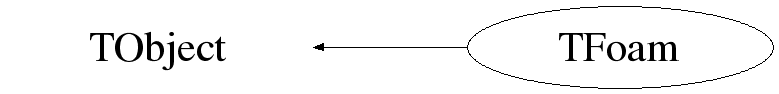## Function documentation

TFoam()
``` Default constructor for streamer, user should not use it.
```
TFoam(const Char_t* Name)
``` User constructor, to be employed by the user
```
~TFoam()
``` Default destructor
cout<<" DESTRUCTOR entered "<<endl;
```
TFoam(const TFoam &From)
``` Copy Constructor  NOT IMPLEMENTED (NEVER USED)
```
void Initialize(TRandom* , TFoamIntegrand* )
``` Basic initialization of FOAM invoked by the user. Mandatory!

This method starts the process of the cell build-up.
User must invoke Initialize with two arguments or Initialize without arguments.
This is done BEFORE generating first MC event and AFTER allocating FOAM object
and reseting (optionally) its internal parameters/switches.
The overall operational scheme of the FOAM is the following:

```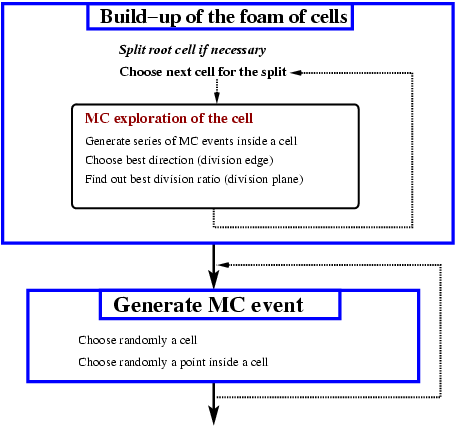```
This method invokes several other methods:

InitCells initializes memory storage for cells and begins exploration process
from the root cell. The empty cells are allocated/filled using  CellFill.
The procedure Grow which loops over cells, picks up the cell with the biggest
``driver integral'', see Comp. Phys. Commun. 152 152 (2003) 55 for explanations,
with the help of PeekMax procedure. The chosen cell is split using Divide.
Subsequently, the procedure Explore called by the Divide
(and by InitCells for the root cell) does the most important
job in the FOAM object build-up: it performs a small MC run for each
newly allocated daughter cell.
Explore calculates how profitable the future split of the cell will be
and defines the optimal cell division geometry with the help of Carver or Varedu
procedures, for maximum weight or variance optimization respectively.
All essential results of the exploration are written into
the explored cell object. At the very end of the foam build-up,
Finally, MakeActiveList is invoked to create a list of pointers to
all active cells, for the purpose of the quick access during the MC generation.
The procedure Explore employs MakeAlpha to generate random coordinates
inside a given cell with the uniform distribution.
The above sequence of the procedure calls is depicted in the following figure:

```void Initialize()
``` Basic initialization of FOAM invoked by the user.
IMPORTANT: Random number generator and the distribution object has to be
provided using SetPseRan and SetRho prior to invoking this initializator!
```
void InitCells()
``` Internal subprogram used by Initialize.
It initializes "root part" of the FOAM of the tree of cells.
```

``` Internal subprogram used by Initialize.
It initializes content of the newly allocated active cell.
```
void Explore(TFoamCell* Cell)
``` Internal subprogram used by Initialize.
It explores newly defined cell with help of special short MC sampling.
As a result, estimates of true and drive volume is defined/determined
Average and dispersion of the weight distribution will is found along
each edge and the best edge (minimum dispersion, best maximum weight)
is memorized for future use.
The optimal division point for eventual future cell division is
determined/recorded. Recorded are also minimum and maximum weight etc.
The volume estimate in all (inactive) parent cells is updated.
Note that links to parents and initial volume = 1/2 parent has to be
already defined prior to calling this routine.
```
void Varedu(Double_t* , Int_t& , Double_t& , Double_t& )
``` Internal subrogram used by Initialize.
In determines the best edge candidate and the position of the cell division plane
in case of the variance reduction for future cell division,
using results of the MC exploration run stored in fHistEdg
```
void Carver(Int_t& , Double_t& , Double_t& )
``` Internal subrogram used by Initialize.
Determines the best edge-candidate and the position of the division plane
for the future cell division, in the case of the optimization of the maximum weight.
It exploits results of the cell MC exploration run stored in fHistEdg.
```
void MakeAlpha()
``` Internal subrogram used by Initialize.
Provides random vector Alpha  0< Alpha(i) < 1
```
void Grow()
``` Internal subrogram used by Initialize.
It grow new cells by the binary division process.
```

``` Internal subprogram used by Initialize.
It finds cell with maximal driver integral for the purpose of the division.
```

``` Internal subrogram used by Initialize.
It divides cell iCell into two daughter cells.
The iCell is retained and tagged as inactive, daughter cells are appended
at the end of the buffer.
New vertex is added to list of vertices.
List of active cells is updated, iCell removed, two daughters added
and their properties set with help of MC sampling (TFoam_Explore)
Returns Code RC=-1 of buffer limit is reached,  fLastCe=fnBuf.
```
void MakeActiveList()
``` Internal subrogram used by Initialize.
It finds out number of active cells fNoAct,
creates list of active cell fCellsAct and primary cumulative fPrimAcu.
They are used during the MC generation to choose randomly an active cell.
```
void ResetPseRan(TRandom* PseRan)
``` User may optionally reset random number generator using this method
Usually it is done when FOAM object is restored from the disk.
IMPORTANT: this method deletes existing  random number generator registered in the FOAM object.
In particular such an object is created by the streamer during the disk-read operation.
```
void SetRho(TFoamIntegrand* Rho)
``` User may use this method to set (register) random number generator used by
the given instance of the FOAM event generator. Note that single r.n. generator
may serve several FOAM objects.
```
void ResetRho(TFoamIntegrand* Rho)
``` User may optionally reset the distribution using this method
Usually it is done when FOAM object is restored from the disk.
IMPORTANT: this method deletes existing  distribution object registered in the FOAM object.
In particular such an object is created by the streamer diring the disk-read operation.
This method is used only in very special cases, because the distribution in most cases
should be "owned" by the FOAM object and should not be replaced by another one after initialization.
```
void SetRhoInt(void* Rho)
``` User may use this to set pointer to the global function (not descending
from TFoamIntegrand) serving as a distribution for FOAM.
It is useful for simple interactive applications.
Note that persistency for FOAM object will not work in the case of such
a distribution.
```

``` Internal subprogram.
Evaluates distribution to be generated.
```
void GenerCel2(TFoamCell*& )
``` Internal subprogram.
Return randomly chosen active cell with probability equal to its
contribution into total driver integral using interpolation search.
```
void MakeEvent(void)
``` User subprogram.
It generates randomly point/vector according to user-defined distribution.
Prior initialization with help of Initialize() is mandatory.
Generated MC point/vector is available using GetMCvect and the MC weight with GetMCwt.
MC point is generated with wt=1 or with variable weight, see OptRej switch.
```
void GetMCvect(Double_t* )
``` User may get generated MC point/vector with help of this method
```

``` User may get weight MC weight using this method
```
void GetMCwt(Double_t& )
``` User may get weight MC weight using this method
```
Double_t MCgenerate(Double_t* MCvect)
``` User subprogram which generates MC event and returns MC weight
```
void GetIntegMC(Double_t& , Double_t& )
``` User subprogram.
It provides the value of the integral calculated from the averages of the MC run
May be called after (or during) the MC run.
```
void GetIntNorm(Double_t& , Double_t& )
``` User subprogram.
It returns NORMALIZATION integral to be combined with the average weights
and content of the histograms in order to get proper absolute normalization
of the integrand and distributions.
It can be called after initialization, before or during the MC run.
```
void GetWtParams(Double_t , Double_t& , Double_t& , Double_t& )
``` May be called optionally after the MC run.
Returns various parameters of the MC weight for efficiency evaluation
```
void Finalize(Double_t& , Double_t& )
``` May be called optionally by the user after the MC run.
It provides normalization and also prints some information/statistics on the MC run.
```
void SetInhiDiv(Int_t , Int_t )
``` This can be called before Initialize, after setting kDim
It defines which variables are excluded in the process of the cell division.
For example 'FoamX->SetInhiDiv(1, 1);' inhibits division of y-variable.
The resulting map of cells in 2-dim. case will look as follows:

```void SetXdivPRD(Int_t , Int_t , Double_t* )
``` This should be called before Initialize, after setting  kDim
It predefines values of the cell division for certain variable iDim.
For example setting 3 predefined division lines using:
xDiv=0.30; xDiv=0.40; xDiv=0.65;
FoamX->SetXdivPRD(0,3,xDiv);
results in the following 2-dim. pattern of the cells:

```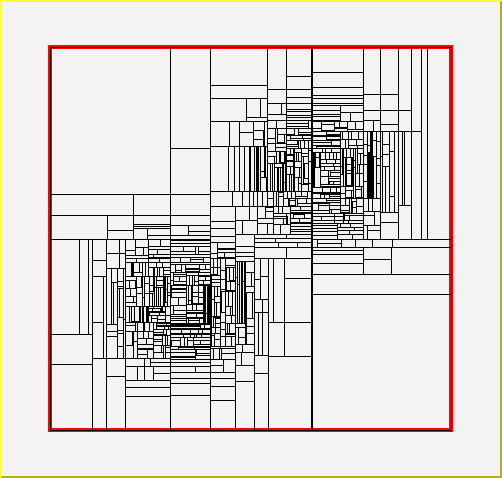void CheckAll(Int_t )
```  User utility, miscellaneous and debug.
Checks all pointers in the tree of cells. This is useful autodiagnostic.
level=0, no printout, failures causes STOP
level=1, printout, failures lead to WARNINGS only
```
void PrintCells(void)
``` Prints geometry of ALL cells of the FOAM
```
void RootPlot2dim(Char_t* )
``` Debugging tool which plots 2-dimensional cells as rectangles
in C++ format readable for root
```
``` Void function for backward compatibility
```

`{return fRho;}`

`{return fPseRan;}`
void SetPseRan(TRandom* PseRan)
`{fPseRan=PseRan;}`
void SetkDim(Int_t kDim)
``` Getters and Setters
```
`{fDim = kDim;}`
void SetnCells(Long_t nCells)
`{fNCells =nCells;}`
void SetnSampl(Long_t nSampl)
`{fNSampl =nSampl;}`
void SetnBin(Int_t nBin)
`{fNBin = nBin;}`
void SetChat(Int_t Chat)
`{fChat = Chat;}`
void SetOptRej(Int_t OptRej)
`{fOptRej =OptRej;}`
void SetOptDrive(Int_t OptDrive)
`{fOptDrive =OptDrive;}`
void SetEvPerBin(Int_t EvPerBin)
`{fEvPerBin =EvPerBin;}`
void SetMaxWtRej(Double_t MaxWtRej)
`{fMaxWtRej=MaxWtRej;}`
const char * GetVersion()
``` Getters and Setters
```
`{return fVersion.Data();}`

`{ return fDim;}`
Double_t GetPrimary(Double_t& prime)
`{return fPrime;}`
void GetPrimary(Double_t& prime)
`{prime = fPrime;}`

`{return fNCalls;}`

``` Debug
```
`{return fNEffev;}`
Double_t Sqr(Double_t x) const
``` Inline
```
`{ return x*x;}`